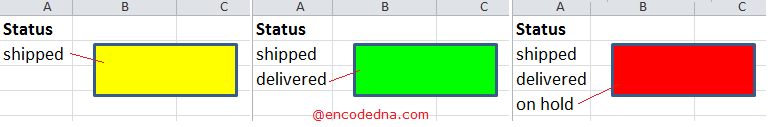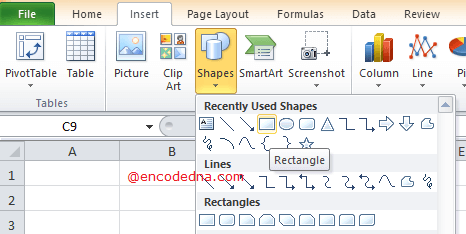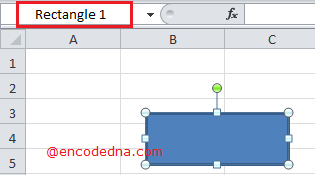﻿ Change the Color of Shape in Excel Based on Cell Value using VBA

# Change the Color of Shape in Excel Based on Cell Value using VBA

You can insert different kinds of shapes in your Excel worksheet, like a circle or a rectangle etc. These shapes can be used for various purposes and you can easily change its properties like the color or the size etc. But, do you know you can dynamically change the color of a shape based on cell value using VBA.

imageLet us assume I have a rectangle shape (just one shape) on my worksheet and I want the macro to change the color of the shape based on certain values entered in the cells. I have a single column named status, which has three rows.

Here are the conditions.

When I enter the value shipped in the second row (A2), the color turns to yellow.
When I enter the value delivered in the third row (A3), the color turns green.
When I enter the value on hold in the fourth row (A4), the color turns red.
When there are no values in either the cells in all the 3 rows, the shape’s color would be white.

So, let's do it.

Open an Excel file and insert a rectangle shape in your worksheet. You can set the default color as white.You may like this: How to add text to Shapes dynamically in Excel using VBA

Click Alt+F11 to open the VBA editor. From the Project Explorer double click the Sheet where you have the data. You can also right click the sheet tab and choose View Code.

Write the code inside Worksheet_Change event. Since, I want to change the shape’s color instantly when the value in any cell changes.

```Option Explicit

Private Sub Worksheet_Change(ByVal Target As Range)

ActiveSheet.Shapes("Rectangle 1").Fill.ForeColor.RGB = vbWhite         ' White is the default color.

If UCase(ActiveSheet.Cells(2, 1)) = "SHIPPED" Then
ActiveSheet.Shapes("Rectangle 1").Fill.ForeColor.RGB = vbYellow
End If

If UCase(ActiveSheet.Cells(3, 1)) = "DELIVERED" Then
ActiveSheet.Shapes("Rectangle 1").Fill.ForeColor.RGB = vbGreen
End If

If UCase(ActiveSheet.Cells(4, 1)) = "ON HOLD" Then
ActiveSheet.Shapes("Rectangle 1").Fill.ForeColor.RGB = vbRed
End If

End Sub```

The color of the shape changes according to the values, which I have explained. Now, see this line in the code …

`ActiveSheet.Shapes("Rectangle 1").Fill.ForeColor.RGB = vbWhite`

## The Shapes() function

The function Shapes() takes a parameter, the name of the shape. You can get the name of the shape by clicking on the shape and see its name in the Name Box. You can change the name of the shape right there.Similarly, you can add different shapes like a circle or an arrow, get its name, provide the name to the function and change the shape’s color using the code.

Also, do this: You can change the color of multiple shapes using the example I have shown above. Simply add more shapes to your sheet and provide the names of each shape to the function. Like this …

```ActiveSheet.Shapes("Rectangle 1").Fill.ForeColor.RGB = vbRed      ' For rectangle or square shape.

OR

ActiveSheet.Shapes("Oval 1").Fill.ForeColor.RGB = vbBlue   ' For circle.```

Well, that’s it. Happy coding. 🙂